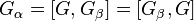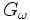# Lower central series

## Definition

The lower central series of a group is a descending series of subgroups indexed by the ordinals. For a group$G$ and an ordinal$\alpha > 0$, the$\alpha^{th}$ member of the lower central series is denoted$\gamma_\alpha(G)$. A more ambiguous notation that may nonetheless be used in some situations for the$\alpha^{th}$ member is$G_\alpha$.

Case for ordinal$\alpha$ Verbal definition of$\alpha^{th}$ member of lower central series Definition using the$\gamma_\alpha(G)$ notation Definition using the$G_\alpha$ notation$\alpha = 1$, i.e., it is the first ordinal the whole group$\gamma_1(G) = G$$G_1 = G$$\alpha$ is a successor ordinal (excluding the case$\alpha = 1$, because we do not define a zeroth member), i.e.,$\alpha = \beta + 1$ for some ordinal$\beta$. Note in particular that this includes the case that$\alpha$ is a positive integer greater than 1. the commutator of two subgroups between the whole group and the$\beta^{th}$ member$\gamma_\alpha(G) = [G,\gamma_\beta(G)] = [\gamma_\beta(G),G]$$G_\alpha = [G,G_\beta] = [G_\beta,G]$$\alpha$ is a limit ordinal the intersection of all previous members$\gamma_\alpha(G) = \bigcap_{\beta < \alpha} \gamma_\beta(G)$$G_\alpha = \bigcap_{\beta < \alpha} G_\beta$

By default, the term is used to refer to only the finite part of the series, i.e. the series$G_n$, for$n \in \mathbb{N}$. This looks like:$G_1 = G \ge G_2 = [G,G] \ge G_3 = [[G,G],G] = [G,[G,G]] \ge G_4 = [[[G,G],G],G] = [G,[G,[G,G]]] = [G,[[G,G],G]] = [[G,[G,G]],G] \ge \dots$

For infinite ordinals, we have:$G_\omega = \bigcap_{n \in \mathbb{N}} G_n, G_{\omega + 1} = [G_\omega,G] = [G,G_\omega], G_{\omega + 2} = [[G_\omega,G],G] = [G,[G,G_\omega]]$

### For a nilpotent group

For a nilpotent group, the lower central series terminates in finitely many steps at the trivial subgroup, and if$G_{c+1}$ is the first member which is trivial, then$G$ is said to have nilpotency class$c$. For a nilpotent group, the lower central series is the fastest descending central series, i.e., if we have a central series:$G = H_1 \ge H_2 \dots H_n = \{ e \}$

Then each$H_i \ge G_i$, and thus,$n \ge c + 1$.

Further information: Lower central series is fastest descending central series

## Facts

### Subgroup properties satisfied by members

Each ordinal gives a subgroup-defining function, namely the ordinal$\alpha$ gives the function sending$G$ to$G_{\alpha}$.$G_1$ is the whole group, while$G_2$ is the derived subgroup (also called the commutator subgroup).

By virtue of each member arising from a subgroup-defining function, it is characteristic. Further, the particular way in which we have made the definitions in fact tells us that all the$G_\alpha$ for finite$\alpha$ are verbal subgroups, while all the$G_\alpha$ (even for infinite$\alpha$ are fully invariant).

There must exist a (possibly infinite) ordinal$\alpha$ such that$G_{\alpha + 1} = G_\alpha$. The subgroup$G_\alpha$ is called the hypocenter of$G$.

### Related group properties

If there is a finite ordinal$c$ for which$G_{c+1}$ is trivial, then$G$ is nilpotent with nilpotency class$c$. The smallest such$c$ is termed the nilpotency class of$G$.

If$G_{\omega}$ is trivial where$\omega$ denotes the first infinite ordinal, then the group is termed residually nilpotent.

If for some infinite ordinal$\alpha$,$G_{\alpha}$ is the trivial group, then$G$ is termed hypocentral.

## Relation with upper central series

For a nilpotent group, the lower central series and upper central series are closely related. They both have the same length, and there is a containment relation between them, which follows from the combination of the facts that upper central series is fastest ascending central series and lower central series is fastest descending central series. However, they need not coincide. Nilpotent groups where they do coincide are termed UL-equivalent groups, and nilpotent not implies UL-equivalent.

Here is a table with some distinctions/contrasts between the two central series:

Nature of fact Fact for lower central series Fact for upper central series
Is the series a strongly central series? Lower central series is strongly central upper central series not is strongly central (i.e., the upper central series need not always be a strongly central series).
What is the nilpotency class of the members of the series? Second half of lower central series of nilpotent group comprises abelian groups, Penultimate term of lower central series is abelian in nilpotent group of class at least three Upper central series may be tight with respect to nilpotency class
Are the members verbal subgroups and/or fully invariant subgroups in the whole group? Lower central series members are verbal (and since verbal implies fully invariant, they are also fully invariant) Upper central series members need not be fully invariant (even for a nilpotent group)

## Relation with derived series

The derived series of a group is a series where each member is defined as the derived subgroup of its predecessor, and the zeroth member is the whole group. The lower central series and derived series are related as follows: the$k^{th}$ member of the derived series is contained in the$2^k$-th member of the lower central series. This follows from the fact that lower central series is strongly central.

## Subgroup series properties

Property Satisfied? Proof Statement with symbols Corollaries
strongly central series Yes Lower central series is strongly central If$m,n$ are natural numbers, then$[G_m, G_n] \le G_{m+n}$. second half of lower central series of nilpotent group comprises abelian groups, nilpotent and every abelian characteristic subgroup is central implies class at most two, derived length is logarithmically bounded by nilpotency class.
strongly characteristic series No Lower central series not is strongly characteristic It is not necessary that a smaller member (with a bigger index) of the lower central series is a characteristic subgroup in every bigger member (with a smaller index), i.e., if$m \ge n$, it is not necessary that$G_m$ is characteristic in$G_n$